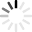# Chemical Formula Quizzes & Trivia

Sitting through a chemistry class can be hard, especially when talking about chemical formulas – which represent elements and compounds as equations. For example, aluminum sulfate has the chemical formula of Al2(SO4)3. You’re probably familiar with H2O, which is two hydrogen molecules and one oxygen molecule, coming together to create water.

Here’s a test – what does the chemical formula of Al(OH)3 create? You know that aluminum is Al on the periodic table, oxygen is O, and hydrogen is H. Put this all together and you’ve got aluminum hydroxide! Chemical formulas can become very complicated, adding in bonds (=) and positive (+) and negative (-) charged molecules. To see how far you’ve come in learning chemical formulas, test your knowledge in one of our chemistry quizzes – they’re bound to create a reaction in your brain!
Top Trending

Revision of Chemical Formulas     This 10-item test will measure how well you understood the rules in writing down  the correct chemical formulas of compounds.  You have 5 minutes to...

Questions: 10  |  Attempts: 7642   |  Last updated: Oct 9, 2020
• Sample Question
Calcium chlorideGives the mind a piece of what daily chemicals are in formulas. Called chemical compounds/formulas.

Questions: 11  |  Attempts: 340   |  Last updated: Mar 9, 2015
• Sample Question
What is the Chemical Formula for Oxygen?Chemistry Form 4 Chapter 2 Chemical Formulae and Equation

Questions: 27  |  Attempts: 85   |  Last updated: Feb 20, 2013
• Sample Question
The proton number of sodium atom is 11 and its molar mass is 23 g/mol. What is the relative atomic mass sodium atom.This quiz is for those of you who would like a 2nd chance at improving your first chemical formula quiz.  Remember, whatever score you receive here will override your first quiz score.

Questions: 20  |  Attempts: 458   |  Last updated: Jan 17, 2013Related